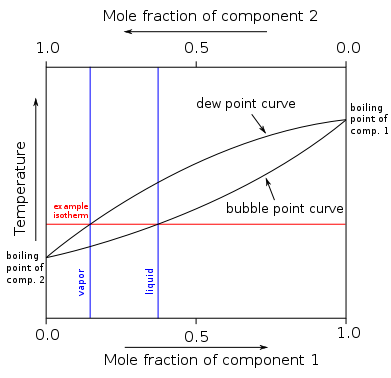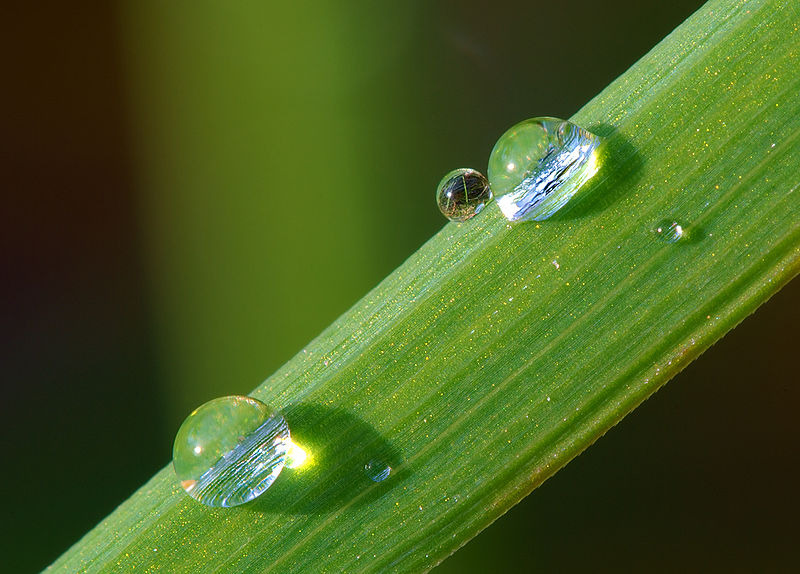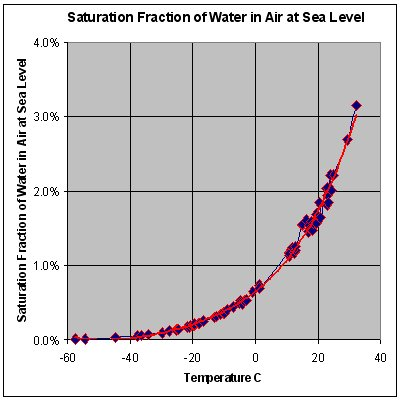# Difference Between Bubble Point and Dew Point

## Main Difference – Bubble Point vs Dew Point

Molecules in a liquid are closely packed but are not tightly packed as in solids. Therefore, attraction forces between these molecules can be broken and converted into its vapor. This is called vaporization. The backward process of this is also possible. This is called condensation. The bubble point and dew point are used to describe this phase change. They refer to temperature values. Both these terms are used in thermodynamics. The bubble point and dew point are very important when designing a distillation system. The main difference between bubble point and dew point is that bubble point is the temperature at which a liquid is formed the first bubble of vapor commencing the vaporization of that liquid whereas dew point is the temperature at which the first drop of dew is formed from vapor commencing the condensation of the liquid.

### Key Areas Covered

1. What is Bubble Point
– Definition, Features, Calculation
2. What is Dew Point
– Definition, Features, Calculation
3. What is the Difference Between Bubble Point and Dew Point
– Comparison of Key Differences

Key Terms: Boiling Point, Bubble Point, Condensation, Dew Point, Humidity, Vapor, Vaporization## What is Bubble Point

The bubble point is the temperature at which a solution makes the first bubble of vapor, commencing the vaporization of that solution (at constant pressure). If it is a pure liquid, then the bubble point is called the boiling point. But if the solution is composed of two or more components, the boiling point of the solution is different from the pure solvent and is called the bubble point.

At the bubble point of a liquid, the ratio between the mole fraction of solute in the vapor phase and the mole fraction of solute in the liquid phase is equal to the distribution coefficient of the liquid at equilibrium.

### Ki   =   yi / xi

Where, Ki is the distribution coefficient

yi is the mole fraction in the vapor phase

xi is the mole fraction in the liquid phaseFigure 1: Curves for Bubble Point and Dew Point

In the above image, the curves for bubble point and dew point are shown. When the mole fraction of one component is zero, and the other component is 1.0, then the bubble point is equal the boiling point of that second component.

## What is Dew Point

The dew point is the temperature at which the vapor of a liquid forms the first bubble of liquid or dew, commencing the condensation of vapor. In other words, the dew point is the temperature to which, vapor should be cooled in order to occur condensation of that vapor.Figure 2:  Dew

Dew point can be measured using a hygrometer. In this equipment, there is a metal mirror that measures the temperature at which the air passes over it is condensed. The term dew point is often described regarding the water vapor. There, the temperature at which liquid water (dew) is formed out of water vapor is measured. Therefore, the dew point has a direct relationship with humidity. If the humidity of air is high, the dew point becomes lower.Figure 3: Dew Point of Water at Sea Level

The above image gives the curve for the temperature at which the air gets saturated with water vapor. After this saturation, the water vapor starts to get condensed. The dew point is calculated using the following equation.

### Td   =   T  –  {(100 – RH) /5}

Where, Td is the dew point

T is the observed temperature

RH is the relative humidity.

The above equation is most suitable when relative humidity is around 50%. Therefore, this is a simple approximation for the calculation of dew point.

## Difference Between Bubble Point and Dew Point

### Definition

Bubble Point: The bubble point is the temperature at which a solution makes the first bubble of vapor, commencing the vaporization of that solution (at constant pressure).

Dew Point: The dew point is the temperature at which the vapor of a liquid forms the first bubble of liquid or dew, commencing the condensation of vapor.

### Phase Change

Bubble Point: The bubble point indicates the temperature at which the liquid phase change into the vapor phase.

Dew Point: The dew point indicates the temperature at which the vapor phase is changed into the liquid phase.

### Applications

Bubble Point: The bubble point can be applied for solutions having at least two components dissolved in it.

Dew Point: The dew point is mostly used regarding water vapor.

### Conclusion

The bubble point and dew point are two terms used to describe special temperatures at which phase changes occur between vapor and its liquid form. The main difference between bubble point and dew point is that bubble point is the temperature at which a liquid forms the first bubble of vapor, commencing the vaporization of that liquid whereas dew point is the temperature at which the first drop of dew forms from vapor, commencing the condensation of a liquid.

##### References:

1. “Introduction to Chemical Engineering Processes/Vapor-Liquid equilibrium.” Introduction to Chemical Engineering Processes/Vapor-Liquid equilibrium – Wikibooks, open books for an open world, Available here. Accessed 16 Aug. 2017.
2. “Dew point.” Wikipedia, Wikimedia Foundation, 15 Aug. 2017, Available here. Accessed 16 Aug. 2017.

##### Image Courtesy:

1. “Binary Boiling Point Diagram new” By Binary_Boiling_Point_Diagram.PNG: H Padleckasderivative work: Michbich (talk) – Binary_Boiling_Point_Diagram.PNG (CC BY-SA 2.5) via Commons Wikimedia
2. “Dew on grass Luc Viatour” By Luc Viatour – Own work www.lucnix.beNikon case D80 optical Sigma 150mm F2,8 Macro and Sigma téléconverter X2 (GFDL) via Commons Wikimedia
3. “Dewpoint” By GregBenson at the English language Wikipedia (CC BY-SA 3.0) via Commons Wikimedia